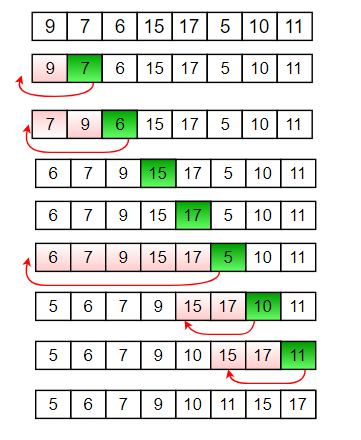# The World of In-Place Sorting AlgorithmsPhoto by Markus Spiske on Unsplash

In Computer Science, sorting algorithms takes an unordered list of numbers and order them in increasing or decreasing order. In-place sorting algorithms moves elements by swapping elements and pushing the remaining elements back by one. We call them in-place algorithms because they do not take up any new space, just rearrange elements within its space.Insertion Sort Algorithm

As illustrated above, sorting algorithms takes an element inside of an array and does some sort of comparison operation to determine if the element is greater or lesser than another element. All sorting algorithms contains comparison operators however, they differ among algorithms. This series will discuss bubble sort, selection sort and insertion sort. Before diving into the algorithms, let’s briefly discuss Big O notation, which is needed to give a thoroughly analysis of the algorithm.

# Big O

What is big O? Big O is an analysis of the algorithm efficiency. Simply put, we are discussing the time it takes for the algorithms to run. When explaining Big O, we discuss the worst case, best case, and average case. Big O Notations include:

1. Constant: O(1); We call this “Big O of 1.” In an interview, a candidate would say, “the algorithm has a time complexity of Big O of 1. “

2. Linear: O(n); We call this “Big of n.” We mostly see this case when for loops are present. In an interview, a candidate would say, “the algorithm has a time complexity of Big O of n” or “it has a quadratic time complexity.”

3. Quadratic: O(n²), We call this “O of N squared.” We usually see this case when a nested for loop are present. In an interview, a candidate would say, “this algorithm has a time complexity of O of N squared or it has a quadratic time complexity.”

# Bubble Sort

Bubble sort sorts items by comparing adjacent elements and swapping if they are out of order, repeating until all items are in sorted order. It continues this process until the list is sorted. Below is an example animation of bubble sort.

As you can see, the algorithm continues to loop through the list until all items are in sorted order. This gives bubble sort an average and worst case time complexity of O(N²). Its best case is when the list is already sorted, which makes the time complexity O(N). Below is an example of bubble sort in python. Try answering the two questions in the comments below.

# Selection Sort

Selection Sort divides an input array into two parts. The left part of the array is the sorted array. This means index zero is the sorted array. It starts with one value. The right side of the array is the unsorted part. This unsorted section is the remaining unsorted items in the original list. The range begins at index 1 to nth number. Below is a visual of the beginning of selection sort. Index 0 is the sorted, the remaining elements are unsorted.

The algorithms begins at index 1, we label this element the minimum element. The algorithm continues to search the unsorted list for an element smaller than the minimum element. It swaps it with the first element that is smaller than the minimum. Then it moves to the next element, label it as the_minimum and continue that process of switching with an element that is smaller than the current index. Below is an example of the selection sort concept.

What makes selection sort unique compared to other sorting algorithms, it makes the minimum number of swaps. The time complexity is quadratic or O(N²). Below is the code for selection sort in python. How can we make this code more optimal? Leave answer in the comments.

# Insertion Sort

Insertion sort begins with the sorted and unsorted approach, index 0 is the sorted and the index 1 to nth number is the unsorted. It iterates over the unsorted list and compares to each element. If the elements is less than current element, it swaps it with the currently element within the sorted list, and inserts it there. The other elements are pushed back as space by one. Below is an example of insertion sort.GeeksforGeeks

The best case for time complexity is O(N) or linear. This will happen if the list is already sorted. The average and worst case case is quadratic.

Software Developer, Change Maker, and all around cool person.